# Statistical terms and methods

The Aboriginal and Torres Strait Islander Health Performance Framework (HPF) uses the following statistical terms and methods for analysis.

### Aboriginal and Torres Strait Islander people and non-Indigenous population descriptors

‘Aboriginal and Torres Strait Islander people’ is the preferred descriptor used throughout the report. The ‘Indigenous Australians’ descriptor is inclusive of all Aboriginal and Torres Strait Islander groups, and is also used where space is limited. The ‘non‑Indigenous Australians’ descriptor is used where the data collection allows for the separate identification of people who are neither Aboriginal nor Torres Strait Islander. The label ‘other Australians’ is used to refer to the combined data for non-Indigenous people, and those for whom Indigenous status was not stated.

### Crude rates

A crude rate is defined as the number of events over a specified period (for example, a year) divided by the total population at risk of the event.

### Age-specific rates

An age-specific rate is defined as the number of events for a specified age group over a specified period (for example, a year) divided by the population of that age group. Age-specific rates are useful for comparing rates across age groups when rates are strongly age-dependent.

### Age-standardisation

This is a method of reducing the influence of age and therefore allowing comparisons of summary rates between two populations that have different age structures. Age-standardisation is used throughout this report when comparing Indigenous Australians with non-Indigenous Australians for a range of variables where age is a factor and when monitoring trends over time.

There are two different methods of age-standardisation, direct and indirect. The method most commonly used for the HPF is direct age-standardisation, however, some tables presenting data from the National Hospital Morbidity Data Collection use the indirect method.

#### Direct age-standardisation

A directly age-standardised rate is defined as the weighted average of age-specific rates, with the weights being equal to the proportion of people in each age group of the standard population. In HPF, the 2001 Australian standard population is used for direct age-standardisation. In general, 5-year age groups up to 75+ are used (0–4, 5–9, …, 70–74 and 75+). However, due to availability and/or size of the data, the 5-year age group may be combined into larger age groups, for example, 10-year age groups, up to 65+ or 55+. In some specific topics, such as smoking during pregnancy, age-standardisation is applied by 5-year age groups for women aged 15 to 44.

#### Indirect age-standardisation

The indirect method is recommended when age-specific numbers of events for the population being studied are not known, or when calculating rates for small populations where fluctuations in age-specific rates can affect the reliability of rates.

### Rate difference and rate ratio

A rate difference is the absolute difference between two rates, and is calculated by subtracting one rate from another. For example, the rate for Indigenous Australians with a particular characteristic minus the rate for non-Indigenous Australians with the same characteristic to show the difference between the two.

A rate ratio measures the relative difference between two population groups and is calculated by dividing one rate (for example, a rate for Indigenous Australians with a particular characteristic) by another (for example, a rate for non‑Indigenous Australians with the same characteristic). A rate ratio greater than 1 indicates a higher rate of the characteristics in the populations of interest, and a ratio of less than 1 suggests a lower rate of the characteristic in the population of interest.

### Standard error (SE) and relative standard error (RSE)

Sample surveys, particularly those conducted by the ABS, are a major source of data for many statistics used in the HPF. The aim of sampling is to achieve ‘representation’ so that the results are the same as if the whole population had been included. When estimates are based on data from a sample that is selected from a population, rather than a full enumeration of that population, they are subject to sampling variability. This means the estimates may differ from the figures that would have been produced if the data had been obtained from the complete population.

Standard error (SE) of the rate measures how accurate the rate estimated from a sample is from its true value. In the HPF, generally the SE for the crude rate is calculated by the following formula: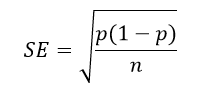where p=m/n, m is the number of incidences and n is the size of the population. When p is very small, for example, the rate of deaths, the 1–p in the above formula can be omitted.

Relative standard error (RSE) is a measure of sampling error which is obtained by expressing the standard error as a percentage of the estimate.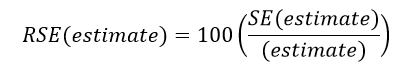In the HPF, we consider that only estimates with a RSE of less than 25%, are sufficiently reliable. Relative standard errors between 25% and 50% should be used with caution. Estimates with a RSE greater than 50% are subject to high sampling error and considered too unreliable for general use.

### Confidence intervals

The observed value of a rate may vary due to chance even where there is no variation in the underlying value of the rate. A confidence interval (CI) is a way to measure the precision of an estimate using standard error (SE). A 95% CI describes a span of numbers around the estimate which has a 95% chance of including the true value. A narrow CI indicates good precision or little random error and, conversely, a wider CI indicates poorer precision. The 95% confidence interval of an estimate p is calculated by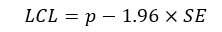and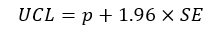where LCL and UCL stand for the lower and upper limit of the confidence interval.

### Annual change and per cent change over time

To use the information from all years available (rather than only the first and last year in a series), linear regression (based on ‘least squares’ method) is used to calculate the annual change and the per cent change over time. The ‘slope’ estimate of the simple linear regression line (Y = a + bX) is used to determine the annual change in the data over the period. The percentage change is calculated taking the difference between the first and last points on the regression line, dividing by the first point on the line and multiplying by 100. In the HPF, these analyses are generally undertaken when there is a minimum of 5 data points, with some exceptions of 4 data points.

### Significance tests

Significance tests are undertaken for data in the HPF to show if the difference between two estimates (for example, between Indigenous and non-Indigenous, between remote and non-remote areas, or between two different years) is different from 0 or significant. Significance tests in HPF are at the level of p < 0.05.

#### The word ‘significant’

Statistically significant differences, for example between jurisdictions or over time, are denoted as ‘significant’. It is important to note, that the term ‘statistically significant’ does not mean practical significance, and should not be used outside its statistical context.

Where results are shown to be statistically significant, an * is placed alongside the statistics included in the data tables. However, not all relationships in the data tables have been tested for significance.

#### Statistical testing for rate differences and rate ratios

The testing hypotheses for rate difference (RD) are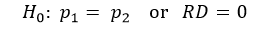and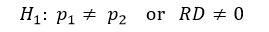where RD = p1 – p2. If RD is outside the interval of (–1.96×SE(RD),1.96×SE(RD)), H0 is rejected and H1 is accepted. In this case we say that p1 and p2 are significantly different at the p < 0.05 level. In other words, the rate difference RD is significantly different from 0 at the p < 0.05 level. Otherwise, if RD is located inside the interval of (-1.96×SE(RD),1.96×SE(RD)), then p1 and p2 are not significantly different at the p < 0.05 level.

The testing hypotheses for rate ratio (RR) are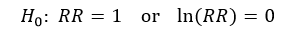andwhere RD = p1 / p2. If ln(RR) is outside the interval of (-1.96 × SE (ln(RR)),1.96 × SE (ln(RR))), H0 is rejected and H1 is accepted. In this case we say that the rate ratio is significantly different from 1 at the p < 0.05 level. Otherwise, the rate ratio is not significantly different from 1 at the p < 0.05 level.

Tables include an * next to the rate ratio and rate difference to indicate that rates for the Indigenous and non-Indigenous populations are statistically different from each other at the p< 0.05 level. Where results of significance testing differ between rate ratios and rate differences, caution should be exercised in the interpretation of the tests.

#### Statistical testing for annual change

The annual change is the coefficient of X in the linear regression model Y = a + bX where X (in years). The testing hypotheses are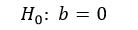and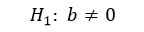b is t-distributed with the degrees of freedom n – 2. Use t* to denote the critical value of t at level 0.05. If b is located outside of the interval (–t* × SE(b), t* × SE(b), then H0 is rejected and H1 is accepted. In this case we say that regression model is significant at the level p< 0.05, or the coefficient b (annual change) is significantly different from 0 at the p < 0.05 level. Otherwise, the annual change is not significantly different from 0 at the p < 0.05 level.

### Suppression of small numbers

In the HPF, small numbers are suppressed due to confidentiality and reliability reasons,

Unless the data provider has a different requirement, the following rules of suppression are applied in the HPF:

1. If the incidence is 4 or less (but not zero), the number and rate will not be reported. The relevant cells will be filled with “n.p.” (not published).
2. If, after suppression, a small number still can be calculated out from the total, another number needs to be supressed. Generally, the rate related to the suppressed cell which is larger than 4 does not need to be supressed, unless the supressed cell can be calculated out from the rate.
3. For direct age-standardised rates, if the incidence is less than 20, the rate will also be replaced by “n.p.”.